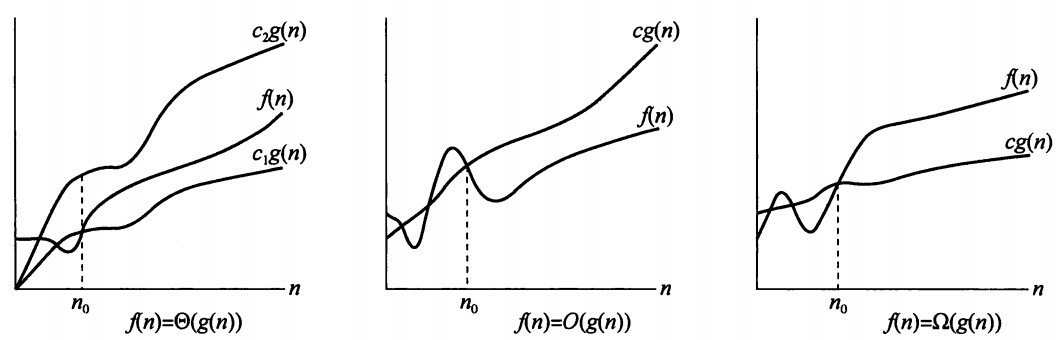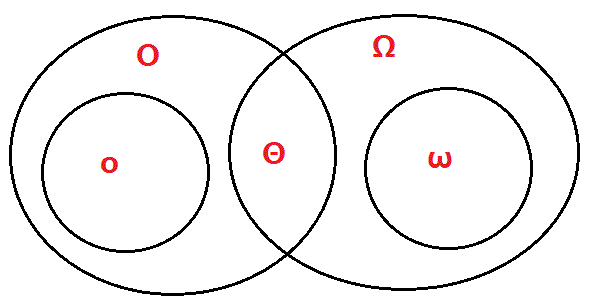# 算法导论------渐近记号Θ、Ο、o、Ω、ω详解

##目录：

## 1.渐近紧确界记号： $Θ$（big-theta）

假设算法A的运行时间表达式$T_1(n)$为：$T_1(n)=30n^4+20n^3+40n^2+46n+100$
假设算法B的运行时间表达式$T_2(n)$为：$T_2(n)=1000n^3+50n^2+78n+10$

于是，算法A的运行时间可以记为：$T_1(n)≈n^4$，记为$T_1(n)=Θ(n^4)$；算法B的运行时间可以记为：$T_2(n)≈n^4$，记为$T_2(n)=Θ(n^4)$

$Θ$的数学含义

由下图中左侧$f(n)=Θ(g(n))$图可以看出，对所有$n>n_0$时，函数$f(n)$乘一个常量因子可等于$g(n)$，我们称$g(n)$$f(n)$的一个 渐近紧确界$Θ$记号在五个记号中，要求是最严格的，因为$g(n)$即可以表示上界也可以表示下界。需要注意的是：$Θ(g(n))$的定义要求每个成员$f(n)∈Θ(g(n))$ 渐近非负，即当n足够大时，$f(n)$非负。 渐近正函数 就是对所有足够大的n均为正的函数。

## 2.渐近上界记号：$O$(big-oh)

根据符号$O$的定义，用它评估算法的复杂度得到的只是问题规模充分大时的一个上界。这个上界的阶越低，评估越精确，越有价值。

$f(n)=O(n^2)$，取$c=2$,$n_0=1$即可
$f(n)=O(n^3)$，取$c=1$,$n_0=2$即可。显然，$O(n^2)$作为上界更为精确。

$O(1)<O(\log(n))<O(n)<O(n\log n)<$$O(n^2)<O(2^n)<O(n!)<O(n^n)$

int isprime(int n) {
for(int i=2; i<=(int)sqrt(n); i++) {
if(n%i==0) {
return0;
}
}
return1;
}


## 3.渐近下界记号：$Ω$(big-omege)

根据符号$Ω$的定义，用它评估算法的复杂度得到的只是问题规模充分大时的一个下界。这个下界的阶越高，评估越精确，越有价值。

$f(n)=Ω(n^2)$，取$c=1$,$n_0=1$即可
$f(n)=Ω(100n)$，取$c=1/100$ ,$n_0=1$即可

>定义1：设$f(n)和g(n)$是定义域为自然数集$N$上的函数。若对于任意正数$c，都存在n_0$，使得对一切$n≥n_0$都有$0≤f(n) $O$记号提供的渐近上界可能是渐近紧确的，也可能是非紧确的。（如：$2n^2=O(n^2)$是渐近紧确的，而$2n=O(n^2)$是非紧确上界。） 例子：$f(n)=n^2+n$，则$f(n)=o(n^3)$ ## 5.非渐近紧确下界：ω(小-omege) >定义1：设$f(n)和g(n)$是定义域为自然数集$N$上的函数。若对于任意正数$c，都存在n_0$，使得对一切$n≥n_0$都有$0≤cg(n)

$ω$记号与$Ω$的关系类似于$o和O$记号的关系。我们用$ω$表示一个非渐近紧确的下界。

## 6.渐近记号Θ、Ο、o、Ω、ω关系

(1)Θ（西塔） 紧确界。 相当于"="
(2)O （大欧） 上界。 相当于"<="
(3)o（小欧） 非紧的上界。 相当于"<"
(4)Ω（大欧米伽） 下界。 相当于">="
(5)ω（小欧米伽） 非紧的下界。 相当于">"## 7.参考资料

1.算法导论  殷建平 译   机械工业出版社 2.算法设计与分析 屈婉玲  著 3.算法设计与分析 王秋芬  吕聪颖著